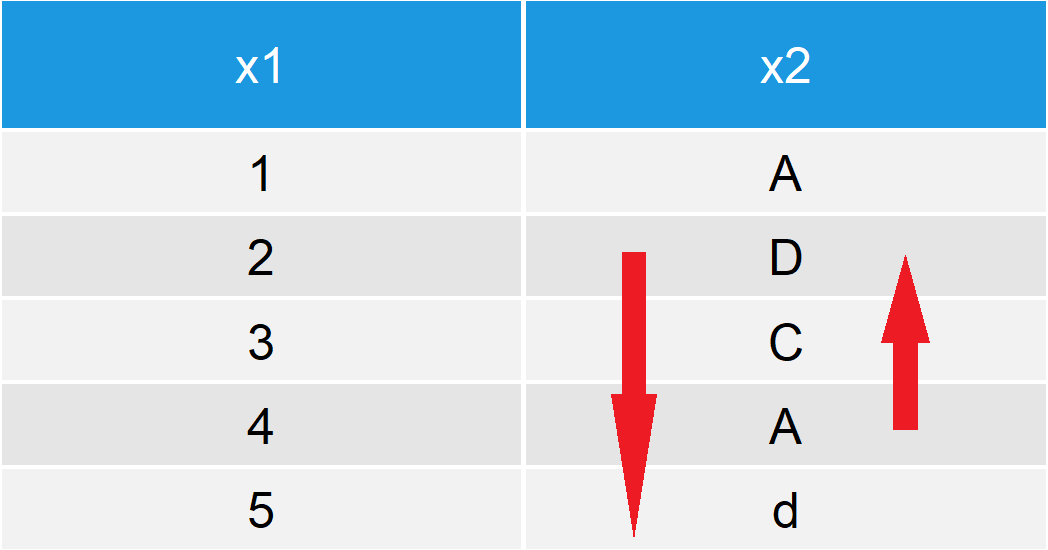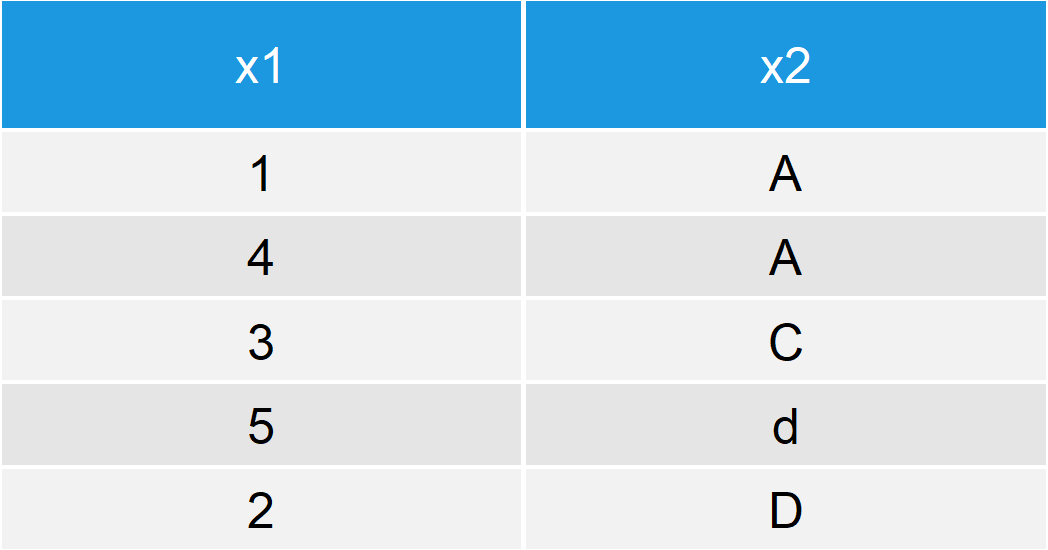# Sort Data Frame in R (4 Examples)

This article explains how to sort a data frame in the R programming language.

I will show you four different examples for the ordering of data frames. More precisely, the tutorial will contain the following contents:

Let’s get started…

## Creation of Example Data

We are going to use the following data set for the examples of this R tutorial:

```data <- data.frame(x1 = 1:5,                         # Create example data
x2 = c("A", "D", "C", "A", "d"))
data                                                 # Print example data```Table 1: Exemplifying Data Frame.

Table 1 shows how our example data frame looks like. Our data contains two columns (i.e. x1 and x2) and five rows.

Table 2 illustrates how our example data should be rearranged, if we want to order it according to the x2 column. The second row should be moved to the bottom and the fourth row should become the second row:Table 2: How Data Frame Should be Ordered.

Let’s do this rearrangement in R…

## Example 1: Sort Data Frame with Base R (order Function)

The Base R installation already provides a good solution for the ordering of our data. We simply need to apply the order function to the column vector according to which we want to sort our data (i.e. x2). Have a look at the following R code:

`data[order(data\$x2), ]                               # Order data with Base R`Table 3: Ordered Data Frame.

As you can see based on Table 3, our data is perfectly ordered in respect to the second column.

However, depending on your personal preferences you might prefer another solution for the sorting of your data. In the following, I’m therefore going to show you some programming alternatives for the ordering of data frames…

## Example 2: Sort Data Frame with dplyr Package (arrange Function)

The dplyr package is a very powerful package for the manipulation of data frames and the package also provides functions for the ordering of a data matrix. Let’s install and load the package to R:

```install.packages("dplyr")                            # Install dplyr R package
library("dplyr")                                     # Load dplyr R package```

Now, we can use the arrange function of the dplyr package as follows:

`arrange(data, x2)                                    # Order data with dplyr`

Have a look at the output of your RStudio console: It will show an ordered data frame in the same way as we sorted it in Example 1.

## Example 3: Sort Data Frame with data.table Package (setorder Function)

Another popular package for the handling of data sets is the data.table package. First, we need to install and load the R add-on package:

```install.packages("data.table")                       # Install data.table R package
library("data.table")                                # Load data.table R package```

In contrast to the previous R codes, the data.table package changes the original data frame automatically. Let’s duplicate our example data in order to retain an original version of the data.

`data_ordered <- data                                 # Replicate example data`

Now, we can sort and print this data frame with the setorder function as follows:

```setorder(data_ordered, x2)                           # Order data with data.table
data_ordered                                         # Print ordered data```

Same output as before – Looks good!

## Example 4: Sort Data Frame in Decreasing Order

So far, we have ordered our data only in increasing order (i.e. from low to high values). However, we can also sort data frames in descending order:

`data[order(data\$x2, decreasing = TRUE), ]            # Order data in decreasing order`

As you can see based on the output of your RStudio console, our example data was ordered alphabetically from high to low letters.

## Video & Further Resources

I have published the examples of this tutorial in a video on the Statistics Globe YouTube channel. You can watch the video below:

Please accept YouTube cookies to play this video. By accepting you will be accessing content from YouTube, a service provided by an external third party.If you accept this notice, your choice will be saved and the page will refresh.

In addition, you might have a look at some of the related R tutorials of my homepage. You can find a selection of tutorials below:

I hope this tutorial taught you how to sort and arrange the rows of a dataframe by column values in the R programming language. In case you have further questions, let me know in the comments.

Subscribe to the Statistics Globe Newsletter